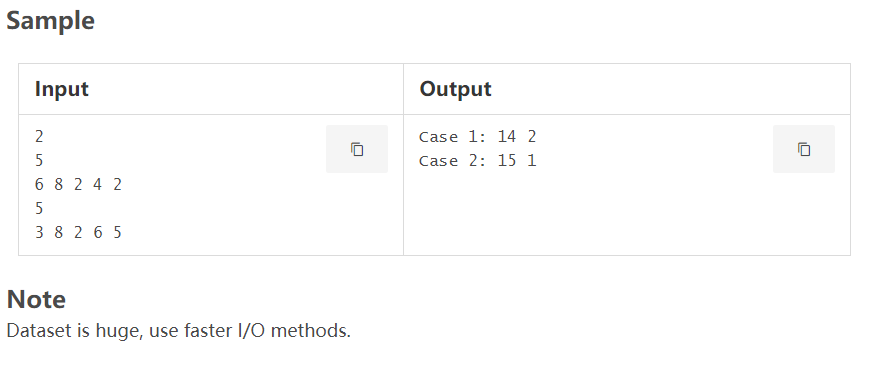# LightOJ 1269 Consecutive Sum(Trie+贪心)

Little Jimmy is learning how to add integers. As in decimal the digits are 0 to 9, it makes a bit hard for him to understand the summation of all pair of digits. Since addition of numbers requires the knowledge of adding digits. So, his mother gave him a software that can convert a decimal integer to its binary and a binary to its corresponding decimal. So, Jimmy's idea is to convert the numbers into binaries, and then he adds them and turns the result back to decimal using the software. It's easy to add in binary, since you only need to know how to add (0, 0), (0, 1), (1, 0), (1, 1). Jimmy doesn't have the idea of carry operation, so he thinks that

• 1 + 1 = 0
• 1 + 0 = 1
• 0 + 1 = 1
• 0 + 0 = 0

Using these operations, he adds the numbers in binary. So, according to his calculations,

3 (011) + 7 (111) = 4 (100)

Now you are given an array of n integers, indexed from 0 to n-1, you have to find two indices i j in the array (0 ≤ i ≤ j < n), such that the summation (according to Jimmy) of all integers between indices i and j in the array, is maximum. And you also have to find two indices, p q in the array (0 ≤ p ≤ q < n), such that the summation (according to Jimmy) of all integers between indices p and *q *in the array, is minimum. You only have to report the maximum and minimum integers.

Input

Input starts with an integer T (≤ 10), denoting the number of test cases.

Each case starts with a line containing an integer n (1 ≤ n ≤ 50000). The next line contains n space separated non-negative integers, denoting the integers of the given array. Each integer fits into a 32 bit signed integer.

Output

For each case, print the case number, the maximum and minimum summation that can be made using Jimmy's addition.题目要求从中找出任意子段，使得他们的异或值最大和最小

利用字典树，由于是任意字段的异或值，利用异或的消去率，将异或前缀放入字典树

const int N=2e6+5;

int n,m;
int i,j,k;
int t[N],tot=0;
int minn,maxx;
int ans;
int a[N];

void init(){ minn=1e9,maxx=0; }

void insert(int x)
{
int res=30;
int rt=0;
while(res!=-1){
int id=(x&(1<<res))?1:0;
if(!t[rt][id]) t[rt][id]=++tot;
rt=t[rt][id];
res--;
}
}

void find(int x)
{
int res=30;
int rt={0,0};
ans=ans=0;
while(res!=-1){
int id=(x&(1<<res))?1:0;
if(t[rt][id]) rt=t[rt][id];
else{
rt=t[rt][id^1];
ans^=(1<<res);
}
if(t[rt][id^1]){
rt=t[rt][id^1];
ans^=(1<<res);
}
else rt=t[rt][id];
res--;
}
}

void clear()
{
for(int i=0;i<=tot;i++) t[i]=t[i]=0;
tot=0;
}

int main()
{
int num=0;
rush(){
sd(n);
init();
insert(0);
for(int i=1;i<=n;i++){
sd(a[i]);
a[i]^=a[i-1];
find(a[i]);
minn=min(minn,ans);
maxx=max(maxx,ans);
insert(a[i]);
}
printf("Case %d: %d %d\n",++num,maxx,minn);
clear();
}
//PAUSE;
}

08-0830708-09564
07-2428
10-301279
06-13160
10-17221
03-121102
11-21264
11-04488
04-07282
07-1853
09-05331
01-271071
01-19688
04-26105
12-26992